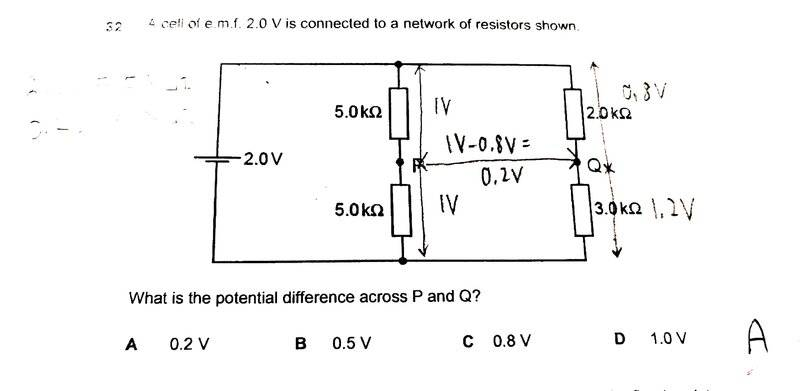# Finding the potential difference between 2 points in a circuit

• ellieee
It would have been nice if you had explained what you did to get the right answer.In summary, the method accepted in this conversation involves splitting 2V equally between the two 5kohm resistors, resulting in 0.8V and 1.2V across the 2kohm and 3kohm resistors, respectively. The potential difference across points P and Q is then calculated as 0.2V. However, this explanation does not include all the necessary steps for a complete understanding.

#### ellieee

Homework Statement
nil
Relevant Equations
nilis this method accepted?
2V is split equally between the 2 5kohms resistor because they are of equal resistance.
2V=5kohms
2kohms= 0.8V
3kohms=1.2V.
p.d across P and Q= 1V-0.8V=0.2V

•Delta2

You've left out a step that one would normally want to include on a test (show HOW you got 1.2v and .8v using current, but certainly you have the right answer and, I think, the right idea.

You have left out two steps in my opinion.

The first step is as indicated by @phinds too at post #3, you need to show why the voltage splits to 0.8 and 1.2V given that the resistors are ##2k\Omega## and ##3k\Omega##.

The second step, which might be scrutiny, however I consider it necessary is if we call O the upper end of resistor of ##5k\Omega## and R the upper end of resistor of ##2k\Omega## and given that ##V_{OP}=1V## and ##V_{RQ}=0.8V## to prove that ##V_{QP}=0.2V##, and given of course that ##V_{OR}=0## since points O and R are connected with a conductor of zero resistance. This step is also related to whether the problem want us to find ##V_{PQ}## or ##V_{QP}##. It turns out that ##V_{PQ}=-0.2V##!

Delta2 said:
why the voltage splits to 0.8 and 1.2V given that the resistors are 2kΩ and 3kΩ.
2V=5kohms
2kohms= 0.8V
3kohms=1.2V.
p.d across P and Q= 1V-0.8V=0.2V
is this explanation not sufficient ?

ellieee said:
2V=5kohms
2kohms= 0.8V
3kohms=1.2V.
p.d across P and Q= 1V-0.8V=0.2V
is this explanation not sufficient ?
It is mostly correct but omits many intermediate steps, outlined in my post #4. If I was your grader I might have cut you up to 40% of the total points.
Tell me how did you calculate that the voltage of 2V splits to 0,8V and 1.2V?

ellieee said:
2V=5kohms
2kohms= 0.8V
3kohms=1.2V.
p.d across P and Q= 1V-0.8V=0.2V
is this explanation not sufficient ?
No, as we keep telling you, you are leaving out steps. Yes, you got the right answer but you could have been guessing for all one can tell from that statement.

•Delta2